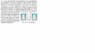# What is the Unknown Temp (T) in TL-TR Equation?

• Miike012

#### Miike012

The Question is in the paint document.

My question to this problem is at the bottom of thread 1

Equation:
T = T3/P3P. Where
T3 is the temperature (K) of water at triple point
I am not 100% sure what P and P3 stand for (can someone tell me)
I believe P is the net pressure (Pressure supplied by the bulb minus the pressure opposing the pressure supplied by the bulb)
and P3 is the pressure at triple point.

Solution:
All Temp are in Kelvin (K)

Define:
TL = Temp of left bulb
PL = Pressure of left bulb
TR = Temp of right bulb
PR = Pressure of right bulb

First Step:

Let TL = T3 and TR = Boiling Point of Water = (BP)

TL - TR = T3 - (BP) =
T3/P3(PL - PR)

Because (TL - TR)<0 Then (PL - PR) = -120torr.

Solving for P3:

P3 =T3(PL - PR)/(T3 - (BP))

Second Step:
Let TL = T3 and TR = Unknown Temp. (T)

TL - TR = T3 - T = T3/P3(PL - PR)

Solving for T:
T = T3 - T3/P3(PL - PR)

QUESTION:
Because I don't know If T>T3 or T<T3 I do not know whether (PL - PR) = +90torr or -90torr.

How am I suppose to know?

#### Attachments

•Temp.jpg
19.1 KB · Views: 374
Last edited:

The Paint document didn't make it thru the posting process. Please repost the attachment.

I figure it out:

Let TL = Temp left bulb
PL = Pressure Left bulb
and
TR = Temp right bulb
PR = Pressure Right bulb

I will keep TR constant at triple point and vary TL:

First Phase: The pressure difference was 120torr when one bulb was at triple point and the other was at boiling point.

If PL - PR = 120then TL>TR

Second Phase: The pressure difference was 90torr when one bbulb was at triple point and the other was at an unknown temperature (T)

If PL - PR = 90 then T>TR